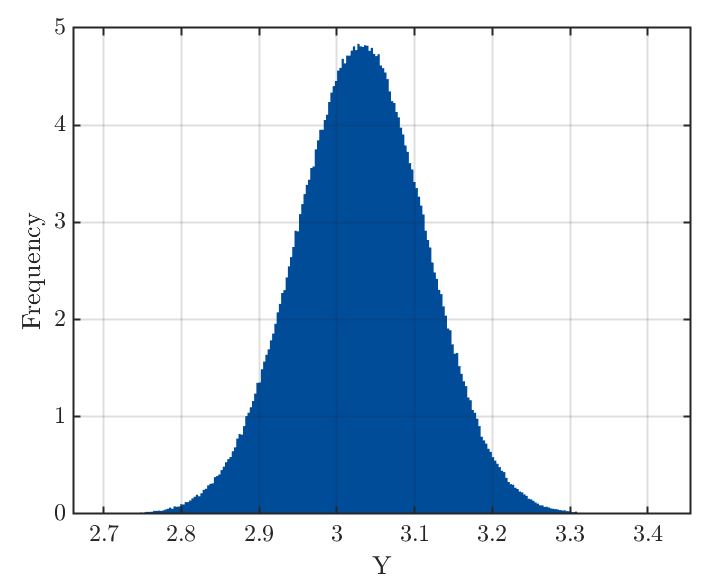# How to perform Monte Carlo simulationbased on built PCE to get histogram and confidence interval of output?

Greeting，the PCE is bulit,then how to perform Monte Carlo simulation based on PCE model to get histogram of output,and store output to print it?

As I have not find the Monte Carlo simulation in PCE tutorial…

PCE is for stochastic system,then if input X is known,then how to compute output Y?

Dear AmosJoseph

You can find an example on how to estimate statistical moments using PCE here:

Best regards,
Styfen

Once the PCE is bulit,we can get statistical moments.

But I ask how to perform Monte Carlo simulation based on the bulit PCE model to get histogram of output,and store the sampling output of Monte Carlo simulation.

Dear AmosJoseph

It’s in fact so straightforward that there is no example on this. We should add it!

Using the example mentioned by @styfen.schaer , once your PCE is built you use the following code:

N_MCS = 10000 ;                  % size of your sample for histogram
XX = uq_getSample(N_MCS);        % sample the inputs from myInput
YY  = uq_evalModel(myPCE,XX);    % evaluate the PCE on the XX sample
hist(YY)


Best regards
Bruno

“”"
myPCE = uq_createModel(MetaOpts);

X = uq_getSample(1e6,‘MC’);
Y = uq_evalModel(myPCE,X);

uq_figure
uq_histogram(Y)
xlabel(’\mathrm Y’)
ylabel(‘Frequency’)
“”"Pardon,I get the Frequency histogram, but the vertical axis is not decimal.
Why?

Dear AmosJoseph,

You simply have to normalize your histogram as explained and demonstrated here:

https://ch.mathworks.com/help/matlab/ref/matlab.graphics.chart.primitive.histogram.html

h = histogram(x,'Normalization','probability')


Best regards,
Styfen

1 Like

By default the histogram created by uq_histogram is already normalized though perhaps not normalized the way you want it. Specifically, the normalization is done such that the area under the histogram is equal to 1. Unless you don’t turn the normalization option off (via named argument 'Normalized'), the y-axis of the histogram produced by uq_histogram is not frequency (i.e., not raw counts). If the area under the curve must be 1 (representing a PDF), then the values at the y-axis do not need to be less than 1.
As already pointed out by @styfen.schaer, the Matlab function histogram does provide several other normalizations not available in uq_histogram. The 'pdf' normalization in histogram is the same as the default normalization of uq_histogram. Note that the 'probability' normalization simply normalizes the histogram with the total number of data points and the area under the histogram won’t be, in general, summed to 1.
I hope this clarifies a bit how uq_histogram work!